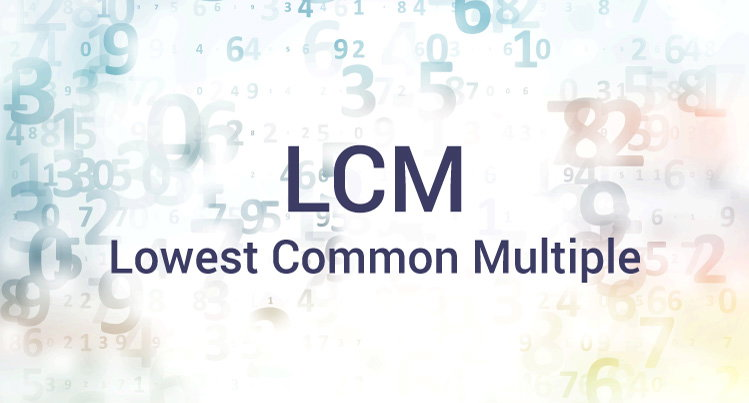# C Program to Find LCM of two Numbers

Examples on different ways to calculate the LCM (Lowest Common Multiple) of two integers using loops and decision making statements.To understand this example, you should have the knowledge of following C programming topics:

The LCM of two integers n1 and n2 is the smallest positive integer that is perfectly divisible by both n1 and n2 (without a remainder). For example: the LCM of 72 and 120 is 360.

## Example #1: LCM using while Loop and if Statement

``````
#include <stdio.h>
int main()
{
int n1, n2, minMultiple;
printf("Enter two positive integers: ");
scanf("%d %d", &n1, &n2);

// maximum number between n1 and n2 is stored in minMultiple
minMultiple = (n1>n2) ? n1 : n2;

// Always true
while(1)
{
if( minMultiple%n1==0 && minMultiple%n2==0 )
{
printf("The LCM of %d and %d is %d.", n1, n2,minMultiple);
break;
}
++minMultiple;
}
return 0;
}
``````

Output

```Enter two positive integers: 72
120
The LCM of 72 and 120 is 360.```

In this program, the integers entered by the user are stored in variable n1 and n2 respectively.

The largest number among n1 and n2 is stored in minMultiple. The LCM of two numbers cannot be less than minMultiple.

The test expression of while loop is always true (1). In each iteration, whether minMultiple is perfectly divisible by n1 and n2 is checked. If this test condition is not true, minMultiple is incremented by 1 and the iteration continues until the test expression of if statement is true.

The LCM of two numbers can also be found using the formula:

```LCM = (num1*num2)/GCD
```

Learn more on, how to find the GCD of two numbers in C programming.

## Example #2: LCM Calculation by Finding GCD

``````#include <stdio.h>
int main()
{
int n1, n2, i, gcd, lcm;

printf("Enter two positive integers: ");
scanf("%d %d",&n1,&n2);

for(i=1; i <= n1 && i <= n2; ++i)
{
// Checks if i is factor of both integers
if(n1%i==0 && n2%i==0)
gcd = i;
}

lcm = (n1*n2)/gcd;
printf("The LCM of two numbers %d and %d is %d.", n1, n2, lcm);

return 0;
}
``````